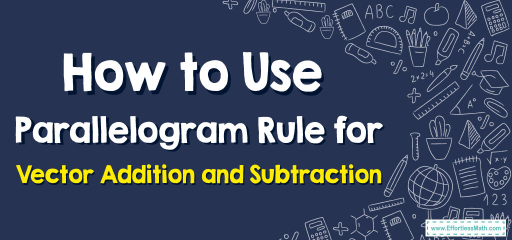# How to Use Parallelogram Rule for Vector Addition and Subtraction

Let's delve into the world of vector operations, specifically focusing on the renowned Parallelogram Rule, a cornerstone in the realm of vector algebra. This guide will be a journey that marries theoretical understanding with vivid geometrical representation.## Step-by-step Guide to Understand Parallelogram Rule for Vector Addition and Subtraction

Here is a step-by-step guide to understanding the parallelogram rule for vector addition and subtraction:

### Preliminary Notions

1. Vectors Defined: Vectors are mathematical entities endowed with both magnitude and direction. They differ from scalar quantities, which possess only magnitude.
2. Parallelogram Visualization: Imagine a parallelogram as a four-sided figure with opposite sides that are equal in length and parallel.

### Chapter I: The Parallelogram Rule for Vector Addition

1. Laying the Groundwork: Given two vectors, $$a$$ and $$b$$, originating from the same initial point, represent them as two adjacent sides of a parallelogram.
2. Construction: Draw the vectors such that their tails coincide. The parallelogram is then constructed using these vectors as consecutive sides.
3. Resultant Vector: The diagonal of the parallelogram that starts from the common tail of the two vectors is the sum (or resultant) of the vectors. Mathematically represented as $$a+b$$, this resultant vector completes the parallelogram.
4. Nuances to Remember: The length (magnitude) of the diagonal represents the magnitude of the resultant vector. Its direction, as indicated by the arrow, showcases the combined effect of vectors $$a$$ and $$b$$.

### Chapter II: The Parallelogram Rule for Vector Subtraction

1. Inception: Vector subtraction can be visualized as the addition of a negative vector. The negative of a vector, $$−b$$, is a vector of the same magnitude as $$b$$ but in the opposite direction.
2. Constructive Visualization: Start by drawing vector $$a$$. Now, from the tail of $$a$$, draw the negative of vector $$b$$, denoted as $$−b$$.
3. Building the Parallelogram: With vectors $$a$$ and $$−b$$ as adjacent sides, construct the parallelogram.
4. Deciphering the Difference: The diagonal starting from the common tail of $$a$$ and $$−b$$ represents the difference between the vectors, given by $$a−b$$. Its magnitude and direction embody the essence of this vector subtraction.

### Epilogue: A Reflective Pause

• The Parallelogram Rule offers a harmonious blend of algebra and geometry, making abstract vector operations palpable and visually graspable.
• As with all mathematical rules, understanding the Parallelogram Rule’s underpinnings and consistently practicing its applications will transform it from a mere rule into an intuitive tool.

May this extensive guide serve as a beacon in your journey to mastering the Parallelogram Rule for vector addition and subtraction. Embrace the complexity, and let the vectors guide your way!

### What people say about "How to Use Parallelogram Rule for Vector Addition and Subtraction - Effortless Math: We Help Students Learn to LOVE Mathematics"?

No one replied yet.

X
30% OFF

Limited time only!

Save Over 30%

SAVE $5 It was$16.99 now it is \$11.99